# Tessellating Regular PolygonsIf we imagine a pearl bracelet projected onto a 2D plane, it looks like a collection of circles arranged, touching, in a larger circle. If, instead of using circles, we used n-sided regular polygons, is it possible to fit these together perfectly (edge-edge) to make a ring, and if so, what is the relationship between the number of sides of each polygon, and the number of polygons that fit into a circle?
Yes, it is possible, for example, here is a bracelet constructed using eighteen regular nonagons (9 sided shapes).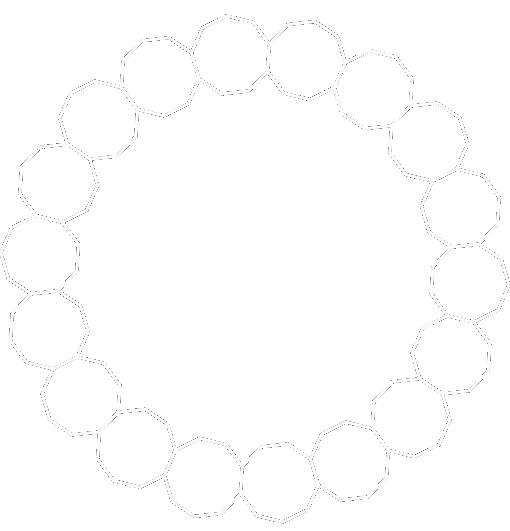Each polygon is touching edge-edge with its adjacent two neighbors.

## Perfect Tessellations

Before we answer the ring question, let’s take a step back and look at perfect tessellations (Euclidean tilings).
Below are representations of the first ten regular polygons; starting with the triangle with three equal sides and angles (and three vertices) through to the Dodecagon with twelve equal sides and angles. Which of these tessellates?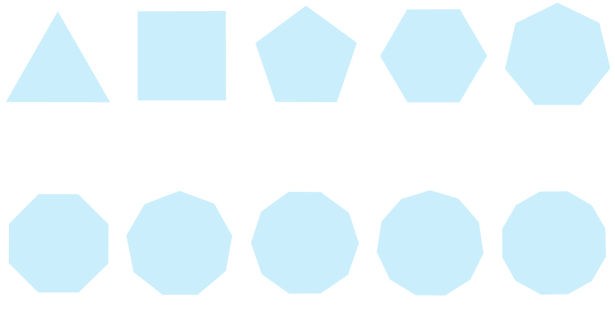Let’s start with the triangle. We can place one on the plane, then add another sharing an edge and common vertex, then another sharing the same vertex, tiling as we go around. After we’ve placed our sixth triangle, we’ve completed an orbit. The last triangle placed fits in perfectly. If these were tiles on the floor they would fit together with no gaps, and be flat on the floor (no bumps). Triangles tesselate on a flat plane.If you know about triangles, you know that all their internal angles add up to 180° As all the angles are equal in regular polygons we know that, for an equilateral triangle, each internal angle is 60°. We can see that there are six triangles that meet at the common vertex and 6 × 60° = 360°, which we know is a complete circle. It’s no surprise that triangles tesselate on a plane.
Similarly, we can do the same with squares. Squares have an internal angle of 90° so we can get four of them (4 × 90° = 360°) around in a circle.A pentagon has five vertices. It does not tessellate.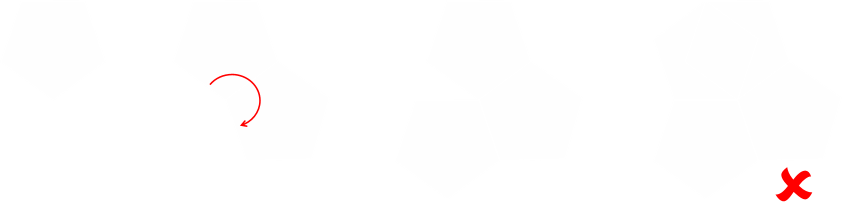Let’s see why. First, we need to calculate the internal angle of a pentagon.
A pentagon can be divided into three triangles. We know that the three angles of a triangle add up to 180°, so the sum of all the angles in the pentagon is 3 × 180° = 540°, and since there are five equal angles in a regular pentagon, each internal angle is 540° ÷ 5 = 108°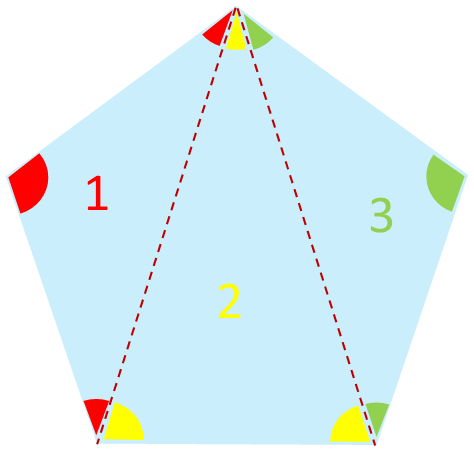Three lots of 108° is 324°, and so is short of a complete circle. Four lots of 108° is 432° which is over a complete circle. Three regular pentagons is too small, four regular pentagons too large. There is no Goldilocks (integer) number of regular pentagons to make a perfect tessellation.
For hexagons, these tesselate. The internal angle for a hexagon is 120°, and it’s easy to compute that three of these fit together in a circle.## Seven, eight, nine, ten …

HeptagonOctagonNonagonDecagon## Are there any more solutions?

There’s a formula for computing the internal angle of a regular polygon, and it’s really simple. Just like pentagon example above, a regular n-sided polygon can be broken up into triangles. For an n-sided shape, it can be broken up into (n-2) triangles. The total of the internal angles is thus (n-2)×180° and, as each angle is equal, the internal angle is (n-2)×180°/n.Here is a table showing a few examples:
SidesTotal AngleInternal Angle
3180°60.000°
4360°90.000°
5540°108.000°
6720°120.000°
7900°128.571°
81,080°135.000°
91,260°140.000°
101,440°144.000°
111,620°147.273°
121,800°150.000°
203,240°162.000°
10017,640°176.400°
1,000179,640°179.640°
10,0001,799,640°179.964°
For a shape to tessllate, the internal angle has to be a factor of 360. Only the triangle, square, and hexagon fit this criterion.

## Proof

Here's a more formal proof. We know, from above, the internal angle of a regular n-sided polygon is (n-2)×180°/n. Let's define the number of times this shape tessellates around as T times. The product of these two needs to be 360°.Simplifying, then adding 4 to each side, and factoring.The only integer solutions to this are {3,6}, {4,4}, and {6,3} corresponding to the triangle, square, and hexagon.
For simple (perfect) tiling, these are the only three solutions.

## Skipping a vertex

There's another class of tessellations we can make if we skip a vertex. Rather than connected the edges directly adjacent, we skip one.Here we can see, for example, that heptagons still do not tessllate around, but now octagons do. Are there other regular polygons that now tessellate?
This tessellation method leaves a hole (which is also a regular polygon) in the middle, and starts us down the path of making our bracelet.
The internal angle of the center hole can be calculated by subtracting two lots of the internal angle of the polygons from a full circle.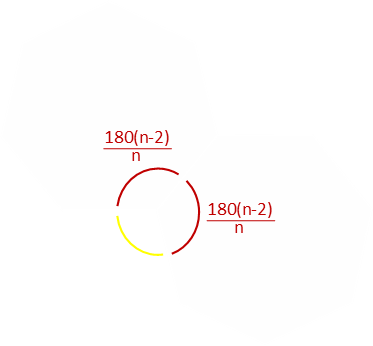For this to tessellate into a ring, the whole in the center needs to be a regular polygon, and so there needs to be a value k such that the internal angle is 180(k-2)/k. Equating these two requirements:If we look for values of n that result in integer solutions for k we can find perfect tessellations. To avoid negative and invalid solutions 5 ≤ n ≤ 12.
nk
510
66
74.667
84
93.600
103.333
113.143
123
We can see from this that the pentagon, hexagon, octagon, and dodecagon tesselate with one skipped vertex. The corresponding holes are shaped decagon, hexagon, square, and triangle.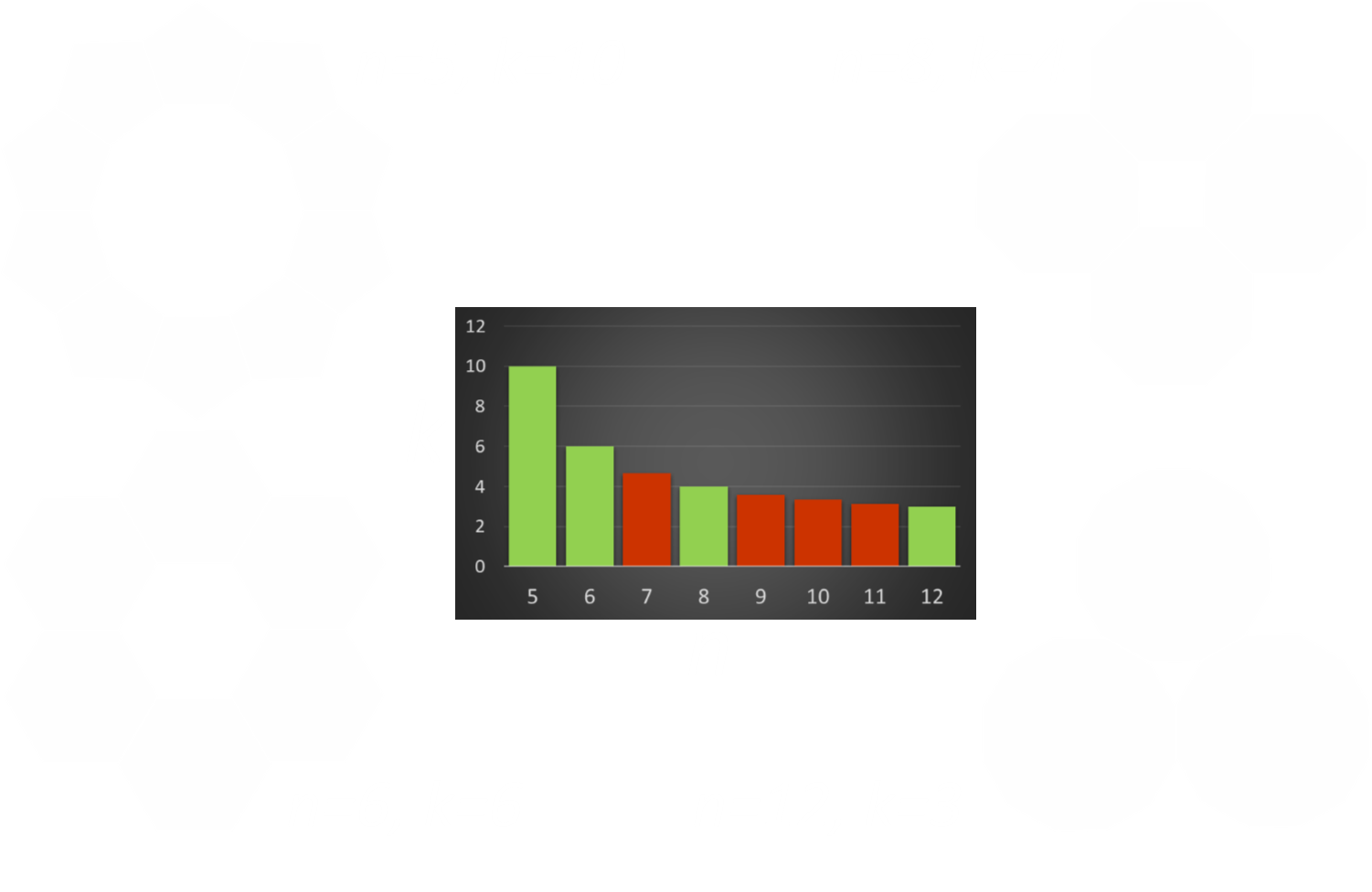## Skipping two vertices

We don't have to stop here. We can skip two vertices. Rather than making a regular polygon shaped center hole, we now have a multipointed star shape in the middle. We can, however, apply the same technique to determine if we can get a perfect tessallation.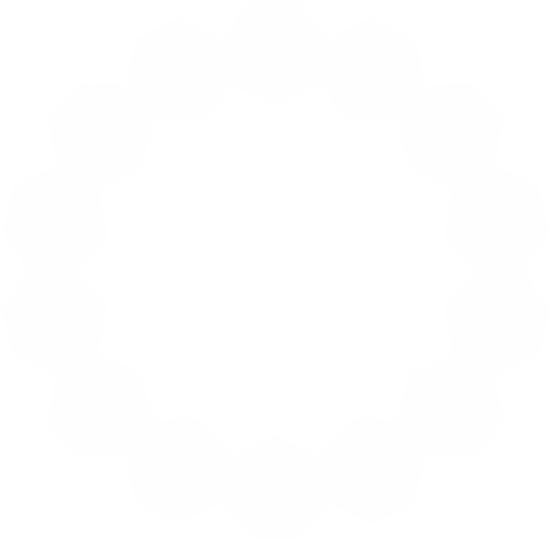A little bit of math gives the relationship between k and n when we skip two verices to be:nk
714
88
96
105
114.400
124
133.714
143.500
153.333
163.200
173.091
183## Skipping three vertices

I think you can see where we are going with this …
Skipping to the third vertex, the hole in the center is looking more like a circular cog wheel, and the relationship is:nk
918
1010
117.333
126
135.200
144.667
154.286
164
173.778
183.600
193.455
203.333
213.231
223.143
233.067
242
Here's one eample: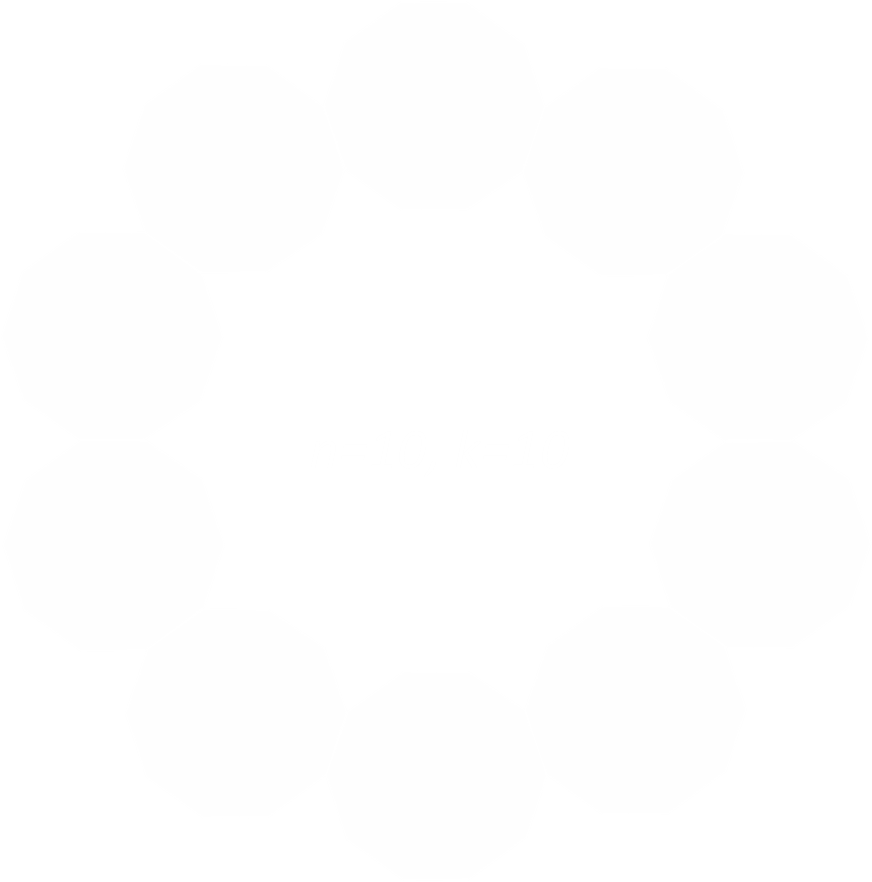## Generic

If we skip m vetices when attempting to make a perfect ring, the solutions that work are the integer solutions to the formula k=2n/(n-2(m+1))